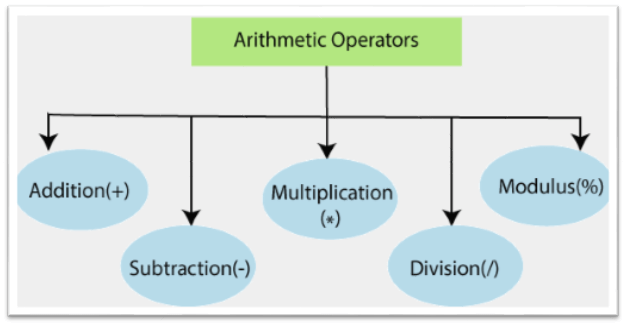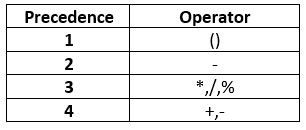# Arithmetic Operators

The arithmetic operators are used in a PowerShell to perform the calculation of the numeric values. By using the arithmetic operators, we can add (+), subtract (-), multiply (*), or divide (/) the values. These operators also include the modulus (%) operator to calculate the remainder of the division operation.

In PowerShell, the addition and multiplication operator may also be used with the strings, hash tables, and arrays. The multiplication (*) operator returns more than one copy of the input, and addition (+) operator concatenates the input.

Windows PowerShell supports the following arithmetic operators:2. – (Subtraction)
3. * (Multiplication)
4. / (Division)
5. % (Modulus)

## Arithmetic Operators Precedence

PowerShell processes these type of operators in expression according to the following precedence rules:The addition operator is an arithmetic operator, which is used to add the two numbers, strings, arrays, and hash tables.

Examples:

Example1: This example adds two numeric values:

The last command will display the value of the variable \$c as 30.

Example2: This example adds two strings:

The last command will display the value of the variable \$z as a single string “PowerShell“.

Example3: This example adds two arrays:

The last command will display the following result of an array \$z:

`1  2  3  A  B  C  `

## Subtraction Operator (-)

The subtraction operator is an arithmetic operator, which is used to subtract one numeric value from another numeric value and make a number to a negative number.

Examples:

Example1: This example subtract one numeric value from other value:

The last command will display the value of the variable \$c as 10.

Example2: This example makes a number to a negative number:

The last command will display the value of the variable \$c as -10.

## Multiplication Operator (*)

The Multiplication operator is an arithmetic operator, which is used to multiply the numeric values or copy the string and array values to the specified number of times.

Examples:

Example1: This example multiplies two numeric values:

The last command will display the value of the variable \$c as 200.

Example2: This example is used to create the copies of the string according to a number which is specified after the multiplication operator:

The last command will display the value of the variable \$y as a single string “ShellShell“.

Example3: This example is used to create the copies of array values according to a number which is specified after the multiplication operator:

The last command will display the following result of an array \$y:

`1  2  3  1  2  3  `

## Division Operator (/)

The Division operator is an arithmetic operator which is used to divide the two numeric values.

The following example divides the two numeric values:

The last command will display the value of the variable \$c as 2.5.

## Modulus Operator (%)

The modulus operator is an arithmetic operator which is used to calculate the remainder of the division operation

The following example calculates the modulus of the two numeric values:

The last command will display the value of the variable \$c as 2.

Previous articleLearn MS Word Tutorial
Next articleWhat is PowerShell cmdlet